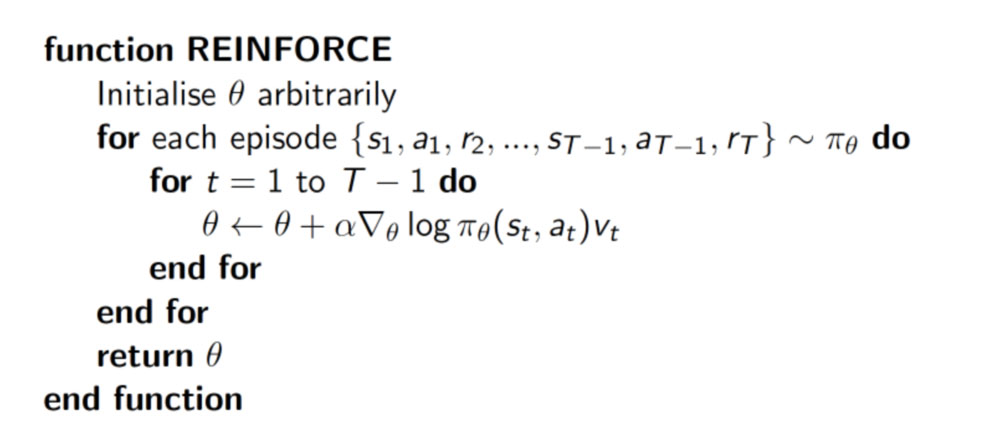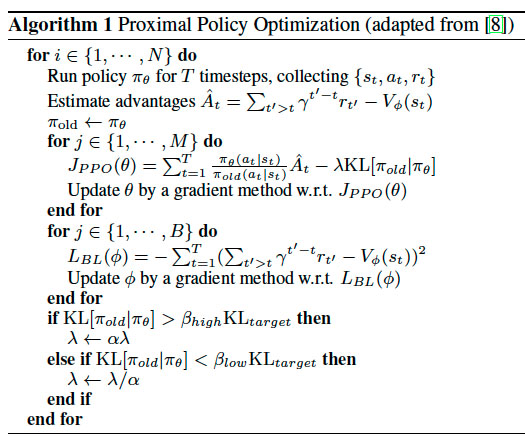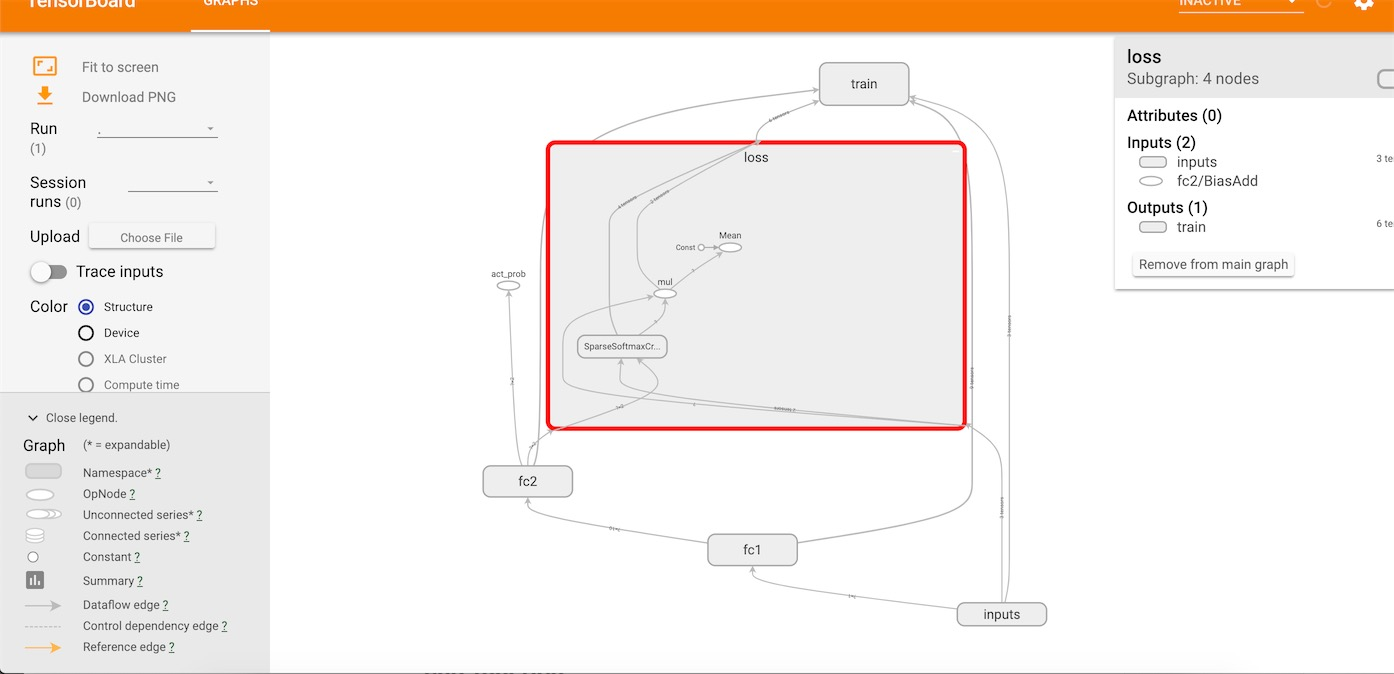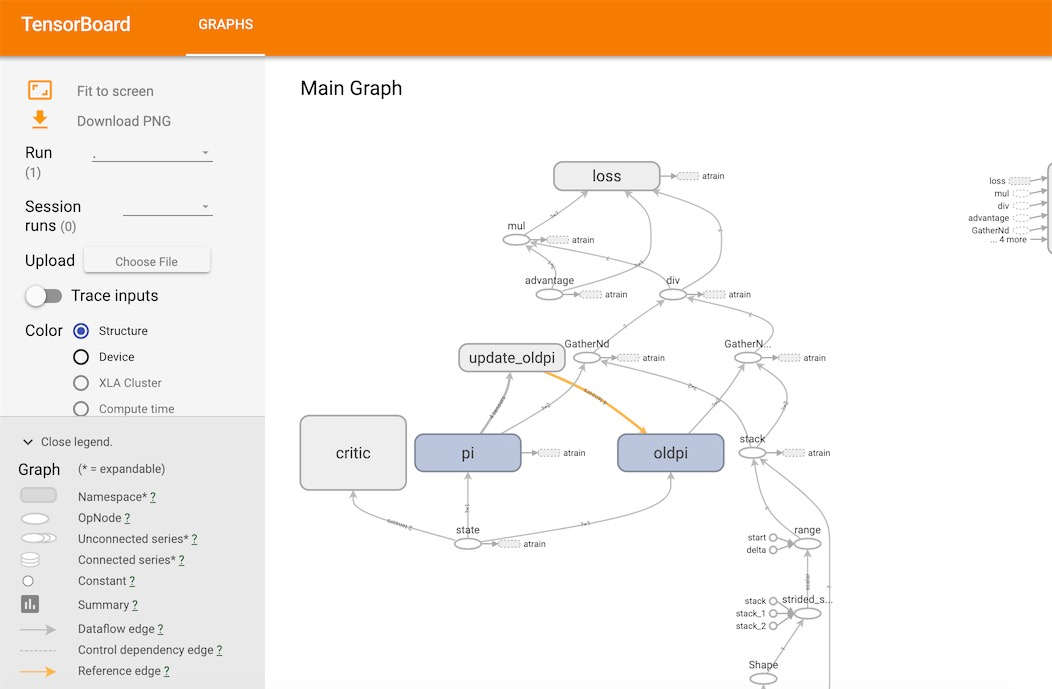## Introduce

• Policy Gradient

Policy gradient 是 RL 中另外一个大家族, 他不像 Value-based 方法 (Q learning, Sarsa), 但他也要接受环境信息 (observation), 不同的是他要输出不是 action 的 value, 而是具体的那一个 action, 这样 policy gradient 就跳过了 value 这个阶段. 而且个人认为 Policy gradient 最大的一个优势是: 输出的这个 action 可以是一个连续的值, 之前我们说到的 value-based 方法输出的都是不连续的值, 然后再选择值最大的 action. 而 policy gradient 可以在一个连续分布上选取 action.

policy gradient 是一种基于 整条回合数据 的更新, 也叫 REINFORCE 方法. 这种方法是 policy gradient 的最基本方法, 有了这个的基础, 我们再来做更高级的。更新网络中的参数，具体的实现方式如下：• PPO

PPO 是 OpenAI 发表的 Trust Region Policy Optimization,基于 Actor-Critic 算法。根据 OpenAI 的官方博客, PPO 已经成为他们在强化学习上的默认算法。PPO 是一套 Actor-Critic 结构, Actor 想最大化 J_PPO, Critic 想最小化 L_BL. Critic 的 loss 好说, 就是减小 TD error. 而 Actor 的就是在 old Policy 上根据 Advantage (TD error) 修改 new Policy, advantage 大的时候, 修改幅度大, 让 new Policy 更可能发生. 而且他们附加了一个 KL Penalty (惩罚项, 不懂的同学搜一下 KL divergence), 简单来说, 如果 new Policy 和 old Policy 差太多, 那 KL divergence 也越大, 我们不希望 new Policy 比 old Policy 差太多, 如果会差太多, 就相当于用了一个大的 Learning rate, 这样是不好的, 难收敛.

## purpose## Algorithm

• PolicyGradient

 with tf.name_scope('loss'):
neg_log_prob = tf.reduce_sum(-tf.log(self.all_act_prob)*tf.one_hot(self.tf_acts, self.n_actions), axis=1)
loss = tf.reduce_mean(neg_log_prob * self.tf_vt)  # reward guided loss


with tf.name_scope('train'):
self.train_op = tf.train.AdamOptimizer(self.lr).minimize(loss)• PPO

#critic
with tf.variable_scope('critic'):
l1 = tf.layers.dense(self.tfs, 100, tf.nn.relu)
self.v = tf.layers.dense(l1, 1)
self.tfdc_r = tf.placeholder(tf.float32, [None, 1], 'discounted_r')
self.advantage = self.tfdc_r - self.v
self.closs = tf.reduce_mean(tf.square(self.advantage))
self.ctrain_op = tf.train.AdamOptimizer(C_LR).minimize(self.closs)

# actor  pi &oldpi
self.pi, pi_params = self._build_anet('pi', trainable=True)
oldpi, oldpi_params = self._build_anet('oldpi', trainable=False)

# actor
def _build_anet(self, name, trainable):
with tf.variable_scope(name):
l_a = tf.layers.dense(self.tfs, 200, tf.nn.relu, trainable=trainable)
a_prob = tf.layers.dense(l_a, A_DIM, tf.nn.softmax, trainable=trainable)
params = tf.get_collection(tf.GraphKeys.GLOBAL_VARIABLES, scope=name)
return a_prob, params



a_indices = tf.stack([tf.range(tf.shape(self.tfa), dtype=tf.int32), self.tfa], axis=1)
pi_prob = tf.gather_nd(params=self.pi, indices=a_indices)   # shape=(None, )
oldpi_prob = tf.gather_nd(params=oldpi, indices=a_indices)  # shape=(None, )
ratio = pi_prob/oldpi_prob
surr = ratio * self.tfadv                       # surrogate loss

with tf.variable_scope('loss'):
self.aloss = -tf.reduce_mean(tf.minimum(        # clipped surrogate objective
surr,
tf.clip_by_value(ratio, 1. - EPSILON, 1. + EPSILON) * self.tfadv))